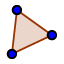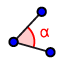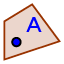# Triangle Interior and Exterior Angles: Quick Exploration

## In the Geometry app below,

1) Use the POLYGONtool to construct triangle ABC. 2) Select the ANGLEtool. Then click anywhere inside the polygon. 3) Go to the Algebra view (click calculator icon upper left). 4) Calculate the sum of the measures of the interior angles of this triangle. (See 0:21 - 1:00 in video at bottom to see how to quickly do this in GeoGebra). ﻿5) Click the MOVEtool. Move A, B, and C. What do you notice?
6) Select the RAYtool. ﻿Construct a ray with endpoint A that passes through B. ﻿ ﻿Construct a ray with endpoint B that passes through C. ﻿ ﻿Construct a ray with endpoint C that passes through A. 7) Select the POINT ON OBJECTtool. ﻿Plot a point D on ray AB that does not lie between A and B. ﻿﻿Plot a point E on ray BC that does not lie between B and C. ﻿ ﻿Plot a point F on ray CA that does not lie between C and A. 8) Select the ANGLEtool again. Then select points D, B, C (in that order). This is one exterior angle of this triangle. 9) Find and display the measures of the other 2 exterior angles of this triangle. 10) Go to the ALGEBRA view. Now calculate the sum of the measures of these 3 exterior angles.

## Question 1

Select the MOVEtool. Move any one (or more) of the triangle's vertices around. What can we conclude about the interior angles of this triangle? Be specific in your response.

## Question 2

Select the MOVEtool. Move any one (or more) of the triangle's vertices around. What can we conclude about the exterior angles of this triangle? Be specific in your response.